# 2023（春）Python程序设计作业1： 基础语法之控制流程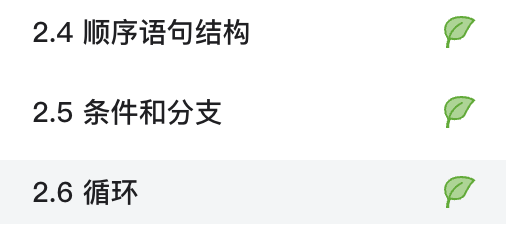## 1.1 顺序结构

`顺序结构`是最简单也是最常见的一种结构，采用顺序结构的程序通常按照由前到后的`顺序执行各个语句`，直到程序结束，结构如下所示：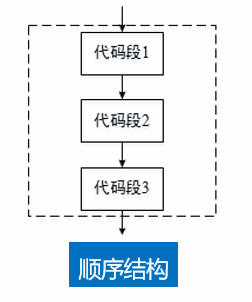## 1.2 顺序结构实例

```
```
1. `a = 2`

2. `b = 3`

3. `c = a + b`

4. `d = c * 24`

5. `print(a, b, c, d)`

输出结果即为：2,3,5,120。

## 2.1 条件表达式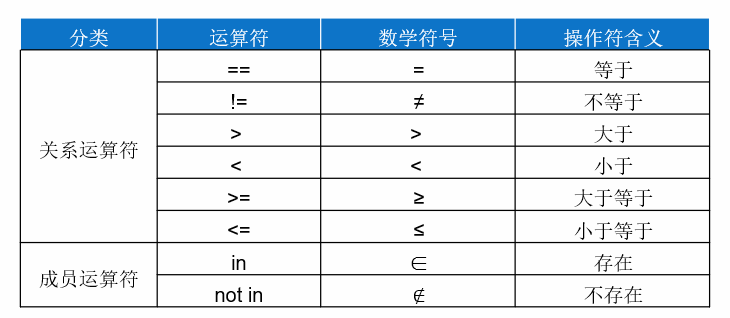Python支持通过保留字not、and和or对判断条件进行逻辑组合：

• not，表示单个条件的“否”关系。如果“条件”的布尔属性为True，“not 条件”的布尔属性就为False；如果“条件”的布尔属性为False，“not 条件”的布尔属性就为True。
• and，表示多个条件之间的“与”关系。当且仅当使用and连接的所有条件的布尔属性都为True时，逻辑表达式的布尔属性为True，否则为False。
• or，表示多个条件之间的“或”关系。当且仅当使用or连接的所有条件的布尔属性都是False时，逻辑表达式的布尔属性为False，否则为True。

## 2.2单分支选择结构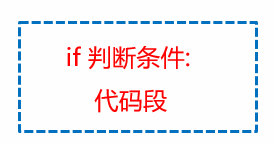• 若if语句中的判断条件成立，执行if语句后的代码段；
• 若判断条件不成立，则跳过if语句后的代码段。
• 单分支结构中的代码段只有“执行”与“跳过”两种情况。

```
```
1. `day = int(input("今天是工作日吗（请输入整数1~7）？"))`

2. `if day in [1,2,3,4,5]:`

3. `print("今天是工作日。")`

4. `if day in [6,7]:`

5. `print("今天非工作日。")`

## 2.3 双分支选择结构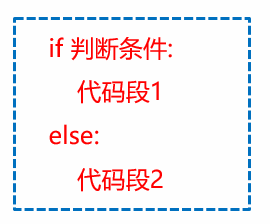• 若if语句中的判断条件成立，执行代码段1
• 若判断条件不成立，则执行代码段2

```
```
1. `day = int(input("今天是工作日吗（请输入整数1~7）？"))`

2. `if day in [1,2,3,4,5]:`

3. `print("今天是工作日。")`

4. `else:`

5. `print("今天非工作日。")`

## 2.4 多分支选择结构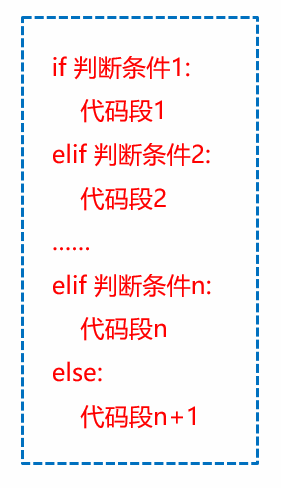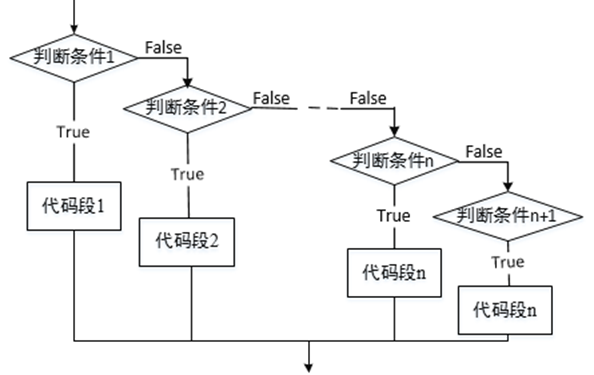## 2.5 选择结构的嵌套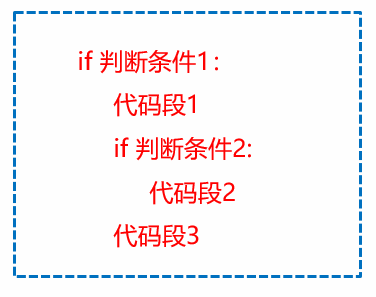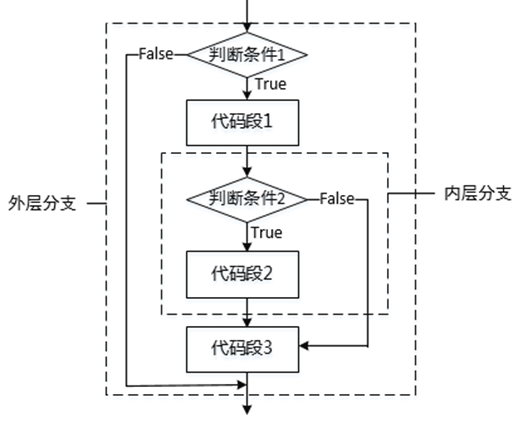## 2.6 三元表达式

python中没有c语言中的三目运算符，但是可以通过以下的形式实现三目运算符的功能

```
```
1. `if x > 0:`

2. `x = x - 1`

3. `else:`

4. `x = x + 1`

```
```
1. `def fun(n):`

2. `return n if n < 2 else fun(n - 1) + fun(n - 2)`

```
```
1. `x = 1`

2. `print([2, 3][bool(x)])`

3. `x = 0`

4. `print([2, 3][bool(x)])`

```
```
1. `3`

2. `2`

# 3 循环语句

while、do while、for

## 3.1 while循环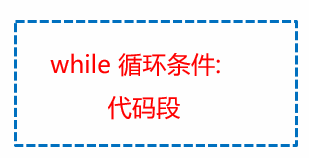• 若循环条件为True，则循环执行while循环中的代码段；
• 若循环条件为False，终止while循环。
• 若while循环的条件总是True，这种情况叫做死循环 。

```
```
1. `n = int(input("请输入一个整数："))`

2. `fact = 1`

3. `i = 1`

4. `while i<= n:`

5. `fact = fact*i`

6. `i = i + 1`

7. `print("n!={}".format(fact))`

## 3.2 while else循环

Python的while循环也支持使用保留字else产生分支。

```
```
1. `n = int(input("请输入一个整数："))`

2. `fact = 1`

3. `i = 1`

4. `print("n!计算中……")`

5. `while i<= n:`

6. `fact = fact*i`

7. `i = i + 1`

8. `else:`

9. `print("n!计算完成 ，循环正常结束")`

10. `print("n!={}".format(fact))`

## 3.3 for 循环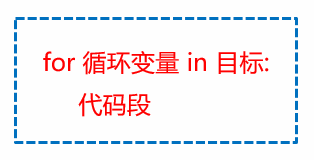• 目标可以是字符串、文件、range()函数或组合数据类型等；
• 循环变量用于保存本次循环中访问到的遍历结构中的元素；
• for循环的循环次数取决于遍历的目标元素个数。

```
```
1. `string = input("请输入一个字符串：")`

2. `for c in string:`

3. `print(c)`

## 3.4 for else循环

for…else循环的具体实现形式：

```
```
1. `for 循环变量 in 目标:`

2. `循环体`

3. `else:`

4. `代码块`

# 4 循环控制语句

·在循环语句中，有时候需要达到中断循环，或者跳过本次循环，执行下次循环的情况，因此就需要有循环控制语句

python中使用break、continue语句控制循环的执行过程

break用于跳出整个循环
continue用于跳出本次循环，继续执行下次循环

## 4.1 break语句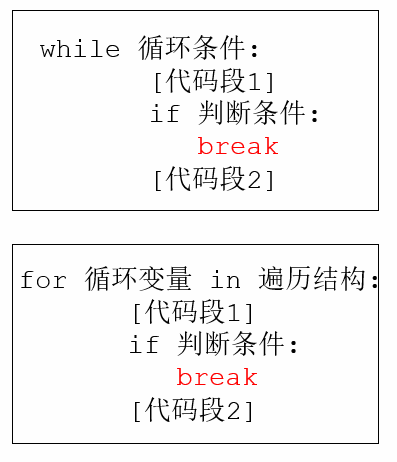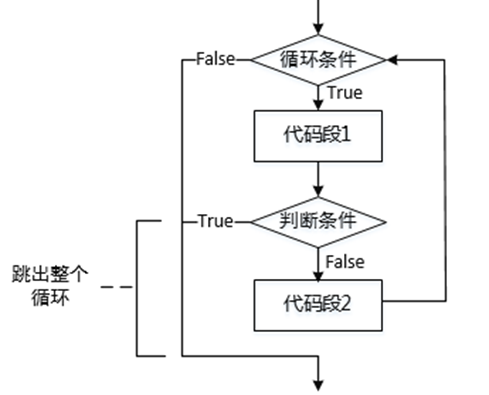## 4.2 continue语句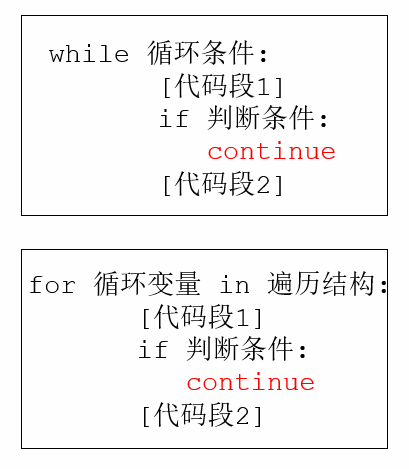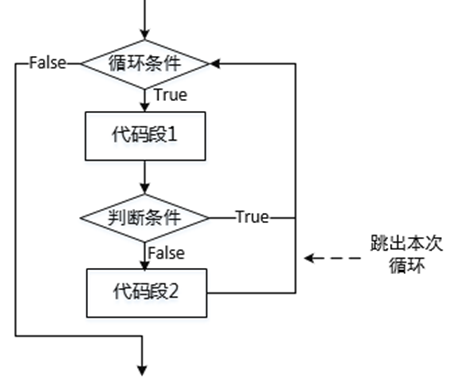## 4.3 pass语句

pass的意思是过，pass掉就是淘汰掉的意思。

...全文
6 回复 打赏 收藏 转发到动态 举报21南开大学《程序设计基础（上）》在线作业参考答案.docx
21南开大学《程序设计基础（上）》在线作业参考答案.docx2023（Python程序设计作业5：Pandas基础技能及综合应用
【代码】2023（Python程序设计作业5：Pandas基础技能及综合应用。2023（Python程序设计作业6：基于unitest的Python测试
【代码】2023（Python程序设计作业6：基于unitest的Python测试。21南开大学《程序设计基础（上）》在线作业-1参考答案.docx
21南开大学《程序设计基础（上）》在线作业-1参考答案.docx2023（Python程序设计作业3： 函数及应用
if y == 0:else:x, y = 4，6二、Lambda函数n = 7三、递归函数if n == 1:return 1else:n = 5。

162发帖与我相关我的任务
python 高校 江苏省·南通市• 近7日
• 近30日
• 至今